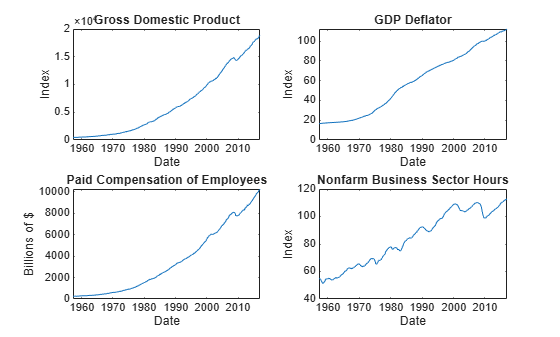# vecm

Convert vector autoregression (VAR) model to vector error-correction (VEC) model

## Syntax

``VECMdl = vecm(Mdl)``

## Description

``VECMdl = vecm(Mdl)` converts the VAR(p) model `Mdl` to its equivalent VEC(p – 1) model representation `VECMdl`.`

## Examples

collapse all

Consider a VAR(2) model for the following seven macroeconomic series.

• Gross domestic product (GDP)

• GDP implicit price deflator

• Paid compensation of employees

• Nonfarm business sector hours of all persons

• Effective federal funds rate

• Personal consumption expenditures

• Gross private domestic investment

Load the `Data_USEconVECModel` data set.

`load Data_USEconVECModel`

For more information on the data set and variables, enter `Description` at the command line.

Determine whether the data needs to be preprocessed by plotting the series on separate plots.

```figure; subplot(2,2,1) plot(FRED.Time,FRED.GDP); title('Gross Domestic Product'); ylabel('Index'); xlabel('Date'); subplot(2,2,2) plot(FRED.Time,FRED.GDPDEF); title('GDP Deflator'); ylabel('Index'); xlabel('Date'); subplot(2,2,3) plot(FRED.Time,FRED.COE); title('Paid Compensation of Employees'); ylabel('Billions of \$'); xlabel('Date'); subplot(2,2,4) plot(FRED.Time,FRED.HOANBS); title('Nonfarm Business Sector Hours'); ylabel('Index'); xlabel('Date');``````figure; subplot(2,2,1) plot(FRED.Time,FRED.FEDFUNDS); title('Federal Funds Rate'); ylabel('Percent'); xlabel('Date'); subplot(2,2,2) plot(FRED.Time,FRED.PCEC); title('Consumption Expenditures'); ylabel('Billions of \$'); xlabel('Date'); subplot(2,2,3) plot(FRED.Time,FRED.GPDI); title('Gross Private Domestic Investment'); ylabel('Billions of \$'); xlabel('Date');```Stabilize all series, except the federal funds rate, by applying the log transform. Scale the resulting series by 100 so that all series are on the same scale.

```FRED.GDP = 100*log(FRED.GDP); FRED.GDPDEF = 100*log(FRED.GDPDEF); FRED.COE = 100*log(FRED.COE); FRED.HOANBS = 100*log(FRED.HOANBS); FRED.PCEC = 100*log(FRED.PCEC); FRED.GPDI = 100*log(FRED.GPDI);```

Create a VAR(2) model using the shorthand syntax. Specify the variable names.

```Mdl = varm(7,2); Mdl.SeriesNames = FRED.Properties.VariableNames;```

`Mdl` is a `varm` model object. All properties containing `NaN` values correspond to parameters to be estimated given data.

Estimate the model using the entire data set and the default options.

`EstMdl = estimate(Mdl,FRED.Variables)`
```EstMdl = varm with properties: Description: "AR-Stationary 7-Dimensional VAR(2) Model" SeriesNames: "GDP" "GDPDEF" "COE" ... and 4 more NumSeries: 7 P: 2 Constant: [15.835 9.91375 -14.0917 ... and 4 more]' AR: {7×7 matrices} at lags [1 2] Trend: [7×1 vector of zeros] Beta: [7×0 matrix] Covariance: [7×7 matrix] ```

`EstMdl` is an estimated `varm` model object. It is fully specified because all parameters have known values.

Convert the estimated VAR(2) model to its equivalent VEC(1) model representation.

`VECMdl = vecm(EstMdl)`
```VECMdl = vecm with properties: Description: "7-Dimensional Rank = 7 VEC(1) Model" SeriesNames: "GDP" "GDPDEF" "COE" ... and 4 more NumSeries: 7 Rank: 7 P: 2 Constant: [15.835 9.91375 -14.0917 ... and 4 more]' Adjustment: [7×7 matrix] Cointegration: [7×7 diagonal matrix] Impact: [7×7 matrix] CointegrationConstant: [7×1 vector of NaNs] CointegrationTrend: [7×1 vector of NaNs] ShortRun: {7×7 matrix} at lag  Trend: [7×1 vector of zeros] Beta: [7×0 matrix] Covariance: [7×7 matrix] ```

`VECMdl` is a `vecm` model object.

## Input Arguments

collapse all

VAR model, specified as a `varm` model object created by `varm` or `estimate`. `Mdl` must be fully specified.

## Output Arguments

collapse all

VEC model equivalent, returned as a `vecm` model object.

## Algorithms

Consider the m-dimensional VAR(p) model in difference equation notation.

`${y}_{t}=c+dt+\sum _{j=1}^{p}{\Gamma }_{j}{y}_{t-j}+\beta {x}_{t}+{\epsilon }_{t}.$`

• yt is an m-by-1 vector of values corresponding to m response variables at time t, where t = 1,...,T.

• c is the overall constant.

• d is the overall time trend coefficient.

• xt is a k-by-1 vector of values corresponding to k exogenous predictor variables.

• β is an m-by-k matrix of regression coefficients.

• εt is an m-by-1 vector of random Gaussian innovations, each with a mean of 0 and collectively an m-by-m covariance matrix Σ. For ts, εt and εs are independent.

• Γj is an m-by-m matrix of autoregressive coefficients.

The equivalent VEC(p – 1) model using lag operator notation is

`$\left(1-L\right){y}_{t}=c+dt+\Pi {y}_{t-1}+\sum _{j=1}^{p-1}{\Phi }_{j}\left(1-L\right){y}_{t-j}+\beta {x}_{t}+{\epsilon }_{t}.$`

• Lyt = yt – 1.

• Π is an m-by-m impact matrix with a rank of r.

• Φj is an m-by-m matrix of short-run coefficients

## Version History

Introduced in R2017b